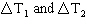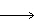Name:    Practice Test 2B

Multiple Choice

1.

How many valence electrons are present in an atom with the following electron configuration?

1s2 2s2 2p6 3s2 3p6 4s2 3d5
 A) 5 B) 7 C) 3 D) 2

2.

What is the energy of one photon of green light with a wavelength of 5200 A° ?
 A) 3.4x10 –30 J B) 3.8x10 –19 J C) 3.8x10 –29 J D) 3.4x10 –40 J

3.

505 J of heat are added to 11.3 g of water at 28.3 °C.  What is the final temperature of water? (Specific heat of water = 4.18 J/g °C)
 A) 17.6 °C B) 47.2 °C C) 39.0 °C D) 32.8 °C

4.

What is DH for the reaction shown below:

N2O3 (g)  ® NO (g)  + NO2 (g)
given the following data:

N2 (g) + 3/2 O2 (g)  ® N2O3 (fg)      DH = 83.7 kJ

N2 (g) +  O2 (g)  ® 2 NO (fg)         DH = 180.4 kJ

1/2 N2 (g) +  O2 (g)  ® NO2 (fg)      DH = 33.2 kJ

 A) 39.7 kJ B) 24.3 kJ C) –207.1 kJ D) 207.1 kJ

5.

Chemist’s favorite station, KMOL, broadcasts at 99.1 MHz.  What is the wavelength of these waves?
 A) 5.33x102 m B) 0.330 m C) 1.88x10–2 m D) 3.03 m

6.

Which of the following atoms has the smallest atomic radius?
 A) Na B) B C) O D) P

7.

Which of the following elements is paramagnetic?
 A) Zn B) Sr C) Kr D) Na

8.

500 mL of 1.0 M NaOH are added to 500 mL of 1.0 M HCl in a Styrofoam cup and the rise in temperature measured as DT1.  The experiment is repeated using 100 mL of each solution and the rise in temperature is measured as DT2.  Which of the following statements aboutis correct?
 A) DT2 is five times as large as DT1 B) DT1 is five times as large as DT2 C) DT1 is equal to DT2 D) No clear statement can be made about DT1 and  DT2

9.

What mass of iron is formed when 725 kJ of heat are released according to the reaction shown below:

2 Al (s) + Fe2O3 (s)2 Fe (s) + Al2O3 (s)              DH = –850 kJ
 A) 130 g B) 95 g C) 65 g D) 47 g

10.

What are the possible number of orbitals in the shell with n= 6?
 A) 16 B) 4 C) 13 D) 36

11.

Which of the following properties, in general, increase as we move left to right across a period in the periodic table?

II)  ionization energy
III) electron affinity
IV) metallic character
 A) II and III B) III and IV C) II only D) I only

12.

What is the maximum number of electrons that the 7th energy level can accomodate?
 A) 8 B) 32 C) 49 D) 98

13.

Which of the following orbitals is the lowest in energy?
 A) 6s B) 5p C) 5d D) 4f

14.

Which of the following correctly lists the elements in order of decreasing IE1?
 A) He > Na > Cl > Ca B) He > Na > Ca > Cl C) He > Cl > Ca > Na D) Ca > Cl > Na > He

15.

The possible values for the magnetic quantum number (ml) for a 5d electron are
 A) 2, 1, 0, –1, –2 B) 3, 2, 1, 0, –1, –2, –3 C) 1, 2, 3, 4 D) 0, 1, 2, 3